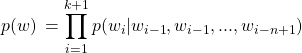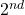# What is the significance of n-grams in a language model ?

n-grams is a term used for a sequence of n consecutive words/tokens/grams.

In general, n-grams can either preserve the ordering or indicate what level of dependency is required in order to simplify the modeling task.

While using bag of Words, n-grams come handy to preserve ordering between words but for language modeling, they signify the independence assumption made in language modeling. Language model can be written asIf we build language model without any order of Markov assumption, above model will have large number of parameters.

For n-gram model, probability of a word depends only on previous n-1 words, i.e.This dependency only on previous n-1 words and not the entire sequence comes with theorder Markov assumption. For bi-gram model, it isorder Markov assumption. One can add “start tokens” to deal with words like